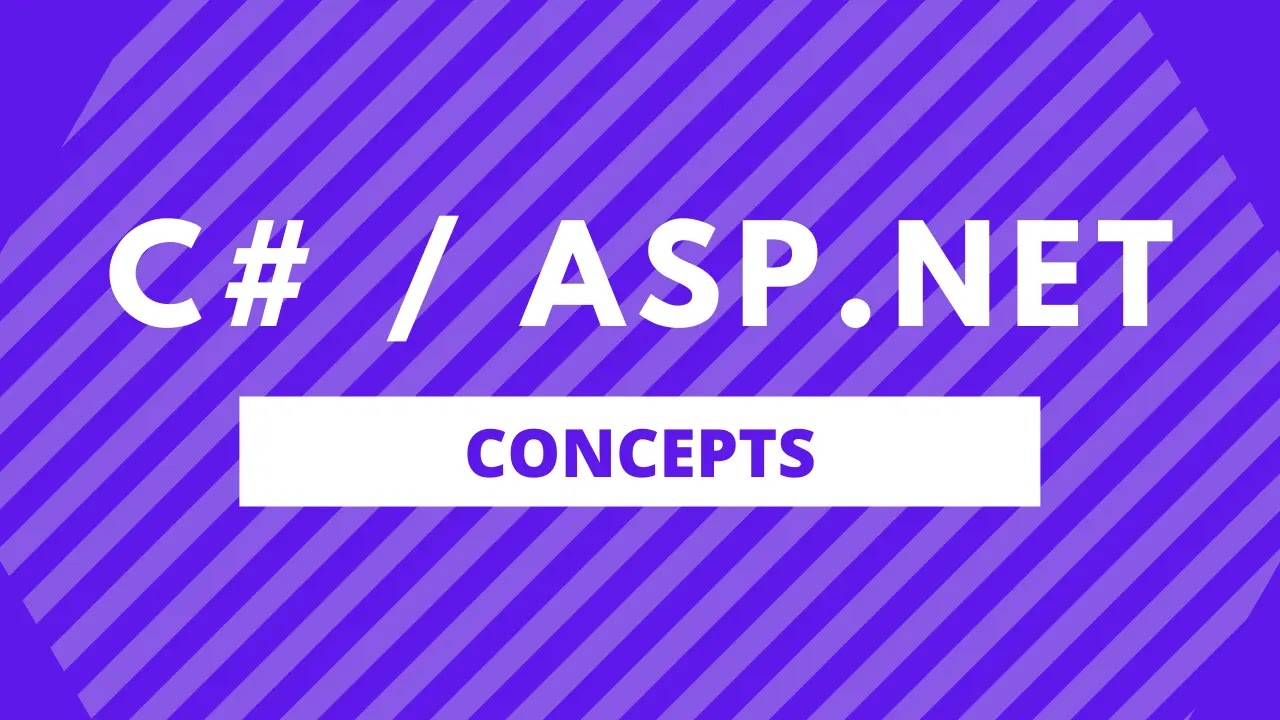# Convert String To Hex In C#

Sameer Saini May 22, 2022In this blog post, we will see how we can convert a string to hex in C#.

We will look at 3 ways by which we can easily convert a string to hex in C#.

## Convert String To Hex In C# Using `Convert.ToHexString()` Method

From .NET 5 onwards, we have the power to use the `Convert.ToHexString()` method that converts an array of 8-bit unsigned integers to its equivalent string representation that is encoded with uppercase hex characters.

This is a really easy way to convert a string to a hex string because C# now provides us a method out of the box.

This method is in the System namespace.

Let's understand this in an example.

Example:

using System; using System.Text; public class Program { public static void Main() { string helloWorld = "Hello World"; byte[] bytes = Encoding.Default.GetBytes(helloWorld); string hexString = Convert.ToHexString(bytes); Console.WriteLine(hexString); } }

Output:

48656C6C6F20576F726C64

In this example, we can see that we converted the string `helloWorld` to a `hexString`, which is a hex string using the `Convert.ToHexString()` method.

## Convert String To Hex In C# Using `BitConverter.ToString()` Method

The BitConverter.ToString() Method converts the numeric value of each element of a specified array of bytes to its equivalent hexadecimal string representation.

We first convert string to be converted to hex into bytes. We then pass these bytes into the `BitConverter.ToString()` method.

This returns us a string with dashes, so the last step is to replace the dahses in the string with empty string.

This method is in the System.Text namespace.

Let's understand this with an example.

Example:

using System; using System.Text; public class Program { public static void Main() { string helloWorld = "Hello World"; byte[] bytes = Encoding.Default.GetBytes(helloWorld); string hexString = BitConverter.ToString(bytes); hexString = hexString.Replace("-", ""); Console.WriteLine(hexString); } }

Output:

48656C6C6F20576F726C64

In this example, we can see that we converted the string `helloWorld` to a `hexString`, which is a hex string with dashes using the `BitConverter.ToString()` method and finally we replaced the dashes with empty string.